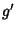## Metric Equivalence Problem

1. Find a complete system of invariants, or

2. decide when two Metrics differ only by a coordinate transformation.
The most common statement of the problem is, ``Given Metricsand, does there exist a coordinate transformation from one to the other?'' Christoffel and Lipschitz (1870) showed how to decide this question for two Riemannian Metrics.

The solution by E. Cartan requires computation of the 10th order Covariant Derivatives. The demonstration was simplified by A. Karlhede using the Tetrad formalism so that only seventh order Covariant Derivatives need be computed. However, in many common cases, the first or second-order Derivatives are Sufficient to answer the question.

References

Karlhede, A. and Lindström, U. ``Finding Space-Time Geometries without Using a Metric.'' Gen. Relativity Gravitation 15, 597-610, 1983.